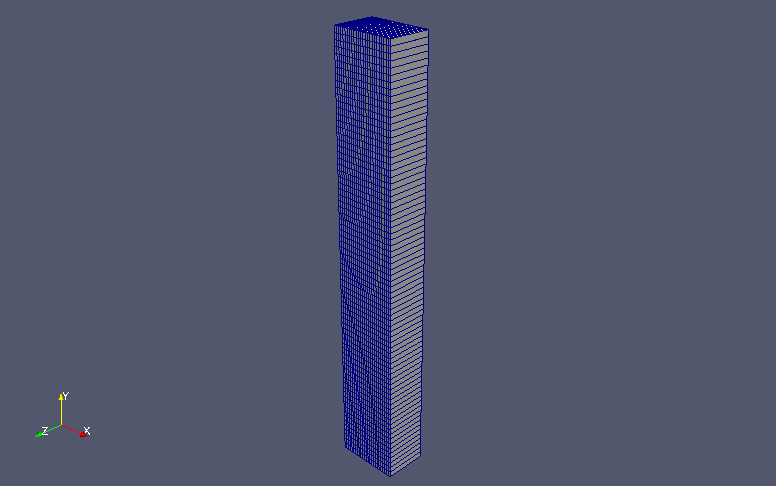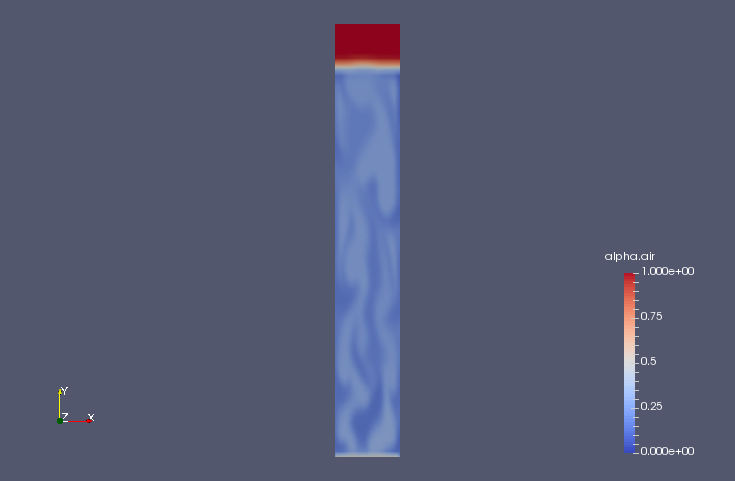﻿ Blowing air into water column (LES) - XSim

Blowing air into water column (LES)

Update: July 28, 2017
OpenFOAM 4.x

Case directory

\$FOAM_TUTORIALS/multiphase/reactingTwoPhaseEulerFoam/LES/bubbleColumn

SummaryModel geometry

We calculate the mixing of air into the water column for 100 seconds. Air flows in from the region "inlet" at the bottom of the water column at (0, 0.1, 0) m/s and out from the region "outlet". We assume that the ratio of water to air is 1:1 in the region "inlet", and the calculation is performed as a two-dimensional problem with one mesh in the z-direction.

The properties of the air and water are set in the files "constant/phaseProperties", "constant/thermophysicalProperties.air", and "constant/thermophysicalProperties.water". In the file "constant/phaseProperties", "type heatAndMomentumTransferTwoPhaseSystem" is also set.

The meshes are as follows, and the number of mesh is 1875.Meshes

The calculation result is as follows.

Volume ratio of air (alpha.air)Volume ratio of air at final time (alpha.air)

Commands

cp -r \$FOAM_TUTORIALS/multiphase/reactingTwoPhaseEulerFoam/LES/bubbleColumn bubbleColumn
cd bubbleColumn

blockMesh

setFields
reactingTwoPhaseEulerFoam

paraFoam

Calculation time

17 minutes 17.88 seconds *Single, Inter(R) Core(TM) i7-2600 CPU @ 3.40GHz 3.40GHz